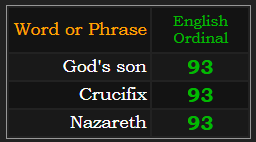Hi, Guest!
Become a Member for an ad-free experience

If I show someone gematria for the first time, I often refer to how the mathematics of the circle are coded into the language.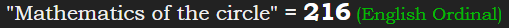### The Moon’s equatorial diameter is 2160 miles216 is 6×6×6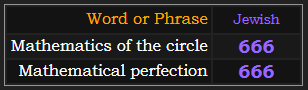Adding 2160 in Roman numerals to the gematria of The Moon is 666.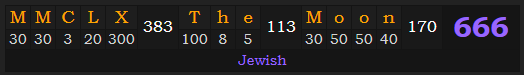All of the numbers in the Magic Square of the Sun sum to 666: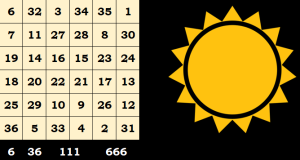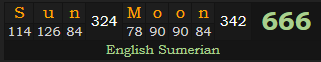The Moon’s average distance from Earth is 238,000 miles.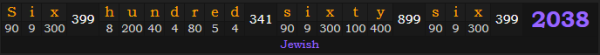The earth’s polar axis is tilted 66.6° from the plane of the sun.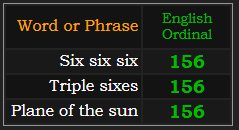An eclipse always occurs either 15, or 6 lunar phases after the most recent one. This is the Hebrew value of eclipse.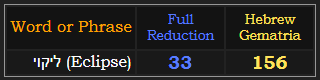Notice the 33 in English Reduction. Thirty-three sums to 156 in Ordinal.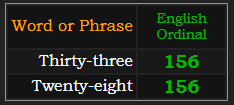I point out the matching gematria of twenty-eight because in Hebrew, the phrase for total solar eclipse sums to 280.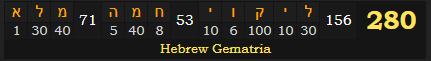28 is a perfect number28 years is exactly 10,227 days: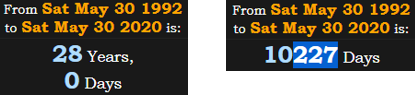227 is the Hebrew value for total eclipse.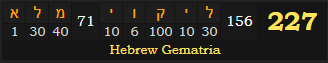(Alt+227) is the code for the Pi symbol on a Windows keyboard. This is because 22÷7 is the smallest equation that gives you the first three digits of pi, 3.14. This equation also sums to 314 in gematria.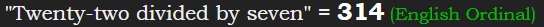There is a cipher built around the number 666 called Satanic Gematria. In that method, eclipse and circles both sum to 314.In English Ordinal, both of these words sum to 69. That’s the Jewish gematria of Pi.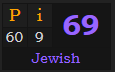### The 314th Prime number is 2083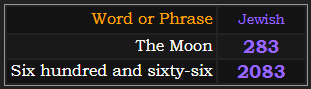The 2017 and 2024 Great American Eclipses are a perfect example of this numerology.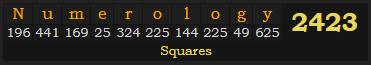Once in a great while, the path of a total solar eclipse will create an X with the path of another recent one. This is what will happen in 2024, when that year’s eclipse will cross paths with the one from 2017: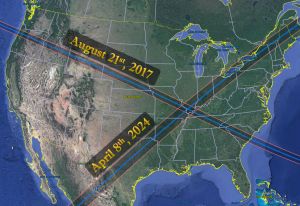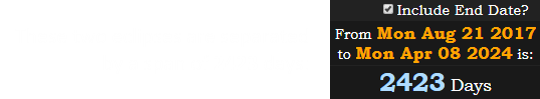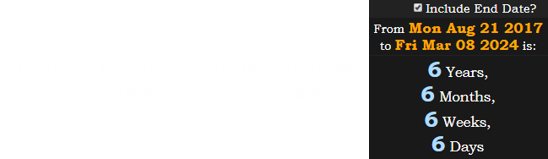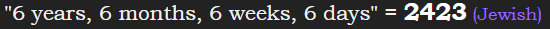### 2423 is the 360th Prime number There are 360 degrees in a circle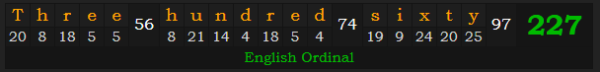Eclipse crucifixion code sums to 227 in the alphabetic order. This is also the Reverse value of Jesus’ crucifixion.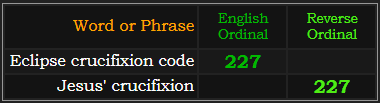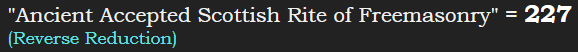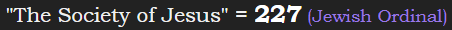The 227th day of the year is written 8/15 or 15/8. The date that leaves 227 days on the calendar is written 5/18 or 18/5: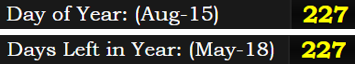### 158 + 185 + 518 + 581 + 815 + 851 = 30187÷22 = 0.318

The second coming is mentioned 318 times in the New Testament.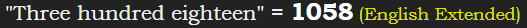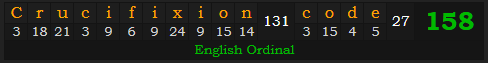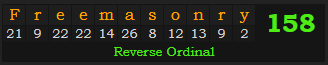The number thirty-two has gematria of 158, as well as 85.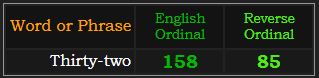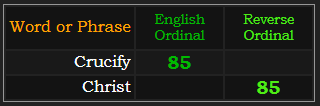Why do I mention 32? This is the Reduction value of Christ.### The 32nd Prime number is 131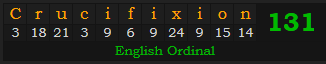The 131st day of the year is May 11th: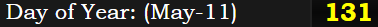You can rearrange those digits to 115, and May 11th is written 11/5. The killing of Christ occurred during Passover.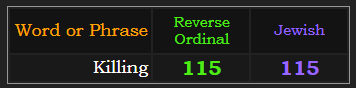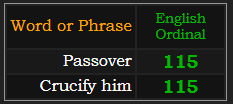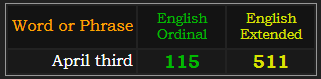Historians believe the Crucifixion of Jesus Christ occurred on April 3rd, 33 A.D., written 4/3/33.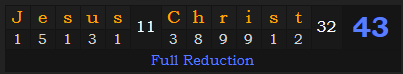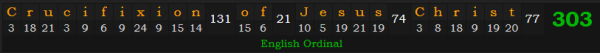This coincides with 16 Nisan on the Hebrew calendar. Nisan is the 7th civil month: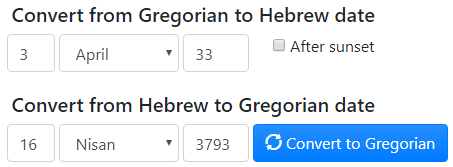16 Nisan would be written as 16/7. “May eleventh” = 167. April 3rd is the 93rd day of the year: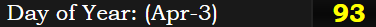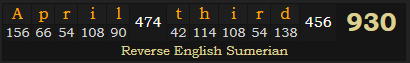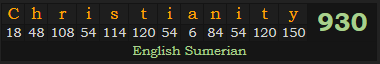The crucifixion occurred between 9 am and 3 pm. The first man, Adam, lived to be 930 years old. 93 is a significant number to Thelemites. The black sun, “Saturn” = 93 and our Sun is an average of 93 million miles from Earth. Jesus is the son of God.